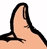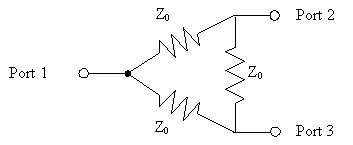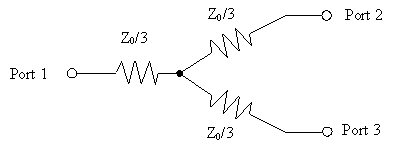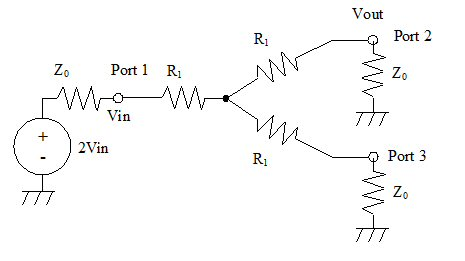# Resistive Power Splitters

Click here to go to our main page on couplers and splitters

New for December 2013: we have information from Andy on additional degrees of freedom with resistive splitters:

We at TEGAM were designing an amplifier test bench and had occasion to look on your site for some splitter design input. I was intrigued with the approach taken by Adams but really wanted to be able to control the attenuation to both output ports. After a bit of fiddling, I came up with a two output port splitter design calculator that lets you set the attenuation to each port (within practical limits). The derivation and calculator are attached in the files names, “Two Output Four DOF”.

In a further step, our design needed a splitter with two equal outputs and a third monitoring output with some “nice” ratio to the others. That was a relatively simple step and is attached as “Three Output Four DOF”.

Go to our download area and grab the Zip file that contains these analyses. Thanks, Andy!

### Wye and Delta equal-split resistive splitters

If you need an unequal split, check out the Owen and the Adams splitters (links above). Use the Owen splitter for maximum isolation, or the Adams splitter for highest efficiency. The Wye and Delta provide equal split.

Resistive power dividers are easy to understand, can be made very compact, and are naturally wideband, working down to zero frequency (DC). Their down side is that a two-way resistive splitter suffers 10xlog(1/2) or 3.0103 dB of real resistive loss , as opposed to a lossless splitter like a hybrid. Accounting for 3.0103 dB real loss and 3.0103 dB power split, the net power transfer loss you will observe from input to one of two outputs is 6.0206 dB for a two-way resistive splitter, so they are often called 6 dB splitters. Dig?

For applications where loss is critical such as power amplifier combiners, the extra loss of a resistive splitter is an unacceptable compromise. But in others, especially in test equipment where power is just an outlet strip away, resistive splitters have their place.

For the 2-way resistive dividers shown below, one half of the power that flows through it is wasted in the resistors. For example, a one watt signal at port 1 will result in two quarter-watt signals at ports 2 and 3 (down by -6 dB). Because of the loss within the network, you have to carefully consider the power dissipation and resistor power ratings. You can put many watts through a "lossless" divider such as a rat-race or branchline coupler. But a watt might burn out a resistive divider. Another disadvantage it the none of the ports are truly isolated from each other.

It's time for a Microwaves101 rule of thumb!The isolation of a resistive splitter is equal to its insertion loss. The 6 dB three-port splitter has (ideally) 6.02 dB loss from any one port to any other port (S21=S31=S23).

The advantages of resistive dividers are size (it can be very small since it contains only lumped elements and not distributed elements), and they can be extremely broadband. Indeed, a resistive power divider is the only splitter that works down to zero frequency (DC). It's so broadband, that we didn't even bother to make a frequency response plot for you!

Below are schematics of the two options for three-port resistive splitters, the "delta" and the "wye". Resistor values as shown will ensure that each port is impedance matched to Z0. These schematics and many others are available in a Word file that you can get in our download area, it's called Electronic_Symbols.doc. You'll find it comes in handy for creating simple block diagrams in Word, PowerPoint or Excel.Delta 6 dB resistive splitterWye 6 dB resistive splitter

New for August 2012: Let's take some time to analyze the wye splitter. First, let's label all of the resistors R1 so we can better keep track of things.

In order for all ports to be matched, the three resistors must be the same, so we gave the value R1.The impedance looking into each port must be matched to Z0. Looking into Port 1, the impedance Zin must equal Z0:

Zin=Z0=R1+(R1+Z0)^2/(2R1+2Z0)

This can be solved pretty quickly to reveal that Z1=Z0/3, with the following advice: first subtract R1 from both sides, them multiply both sides by 2*(R1+Z0). You will soon find out that R1 must equal Z0/3 to match the three ports.

This even better technique for solving for Z0 came from John:

Actually, the solution is even easier than you suggest once you notice that the numerator and the denominator share a factor  (R1 + Z0)

Zin = R1 + (R1 + Z0)^2/(2(R1 + Z0)) = R1 + (1/2)(R1 + Z0) = Z0

Z0 = (3/2) R1 + (1/2) Z0

(1/2) Z0=3/2 (R1)

R1=Z0/3

### N-way resistive splitters (equal split)

You can make N-way resistive splitters easily from the Wye splitter. The Delta resistor becomes a nightmare network for more than a two-way split, it can't be constructed in two dimensions.

The appropriate resistors for an N-port Wye splitter are found by the equation:

R=Z0x(N-1)/(N+1)

For examples, a three-way splitter needs resistors of Z0/2, while a four-way splitter needs resistors of 3xZ0/5, and so on.

The efficiency of a resistive splitter gets worse and worse the more arms you split to. The transferred power ratio is (1/N)^2, as opposed to a lossless splitter that varies as 1/N. So for a four-way splitter, only 1/16 of the power makes it out to one of the four matched loads. It's time for another Microwaves101 rule of thumb!To put it simply, the resistive splitter has double the dB loss compared to a lossless splitter's insertion loss. Thus a two-way resistive splitter transfers -6.02 dB power to each arm, a three-way splitter transfers -9.54 dB, a four-way transfers -12.04 dB, etc. An infinite-way resistive splitter would lose 100% of the incident power and transfer nothing to the loads!

### Fractional dissipation in the Wye resistive splitter

New for February 2007! We've solved this algebra problem for the Wye splitter, here it is. If the resistor closest to the common port is designated "resistor A" and the other two resistors are designated "resistor B" and "resistor C" then:

Power dissipated in resistor A:

PDissA=Pin x (N-1)/(N+1)

Power dissipated in B and C are equal by symmetry.

PDissB=Pin x (N-1)/[(N+1)xN^2)]

Let's tie it all together. The table below shows the fractional dissipation and the output powers. The "power factor" is a measure of the efficiency of the network, it is the sum of all the output powers divided by the input power.

N PDissA PDissB or PdissC Pout Power factor
2 33.3% (-4.77 dB) 8.33% (-10.79 dB) 25% (-6.02 dB) 50% (-3.01 dB)
3 50% (-3.01 dB) 5.56% (-12.55 dB) 11.1% (-9.54 dB) 33.3% (-4.77 dB)
4 60% (-2.22 dB) 3.75% (-14.26 dB) 6.25% (-12.04 dB) 25% (-6.02 dB)
5 66.7% (-1.76 dB) 2.67% (-15.74 dB) 4% (-13.98 dB) 20% (-6.99 dB)
6 71.4% (-1.46 dB) 1.98% (-17.02 dB) 2.78% (-15.56 dB) 16.7% (-7.78 dB)

### Fractional dissipation in N=2 Delta resistive splitter

We only use the delta splitter for N=2, it doesn't make sense for higher order splitters because it becomes a 3D nightmare.

The fractional dissipation in an N=2 delta splitter is easy to calculate. The resistors that are in series with the split ports dissipate as much power as the two outputs. If the series resistors are labeled "resistor A" and the resistor that shunts the output ports is "resistor B", then the dissipation is given by:

N PDissA PDissB Pout Power factor
2 25% (-6.02 dB) 0 25% (-6.02 dB) 50% (-3.01 dB)